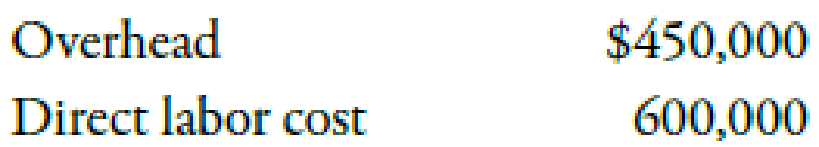Chapter 4, Problem 29BEB### Managerial Accounting: The Corners...

7th Edition
Maryanne M. Mowen + 2 others
ISBN: 9781337115773

#### Solutions

Chapter
Section### Managerial Accounting: The Corners...

7th Edition
Maryanne M. Mowen + 2 others
ISBN: 9781337115773
Textbook Problem
1 views

# Predetermined Overhead Rate, Overhead ApplicationAt the beginning of the year, Estes Company estimated the following costs:Estes uses normal costing and applies overhead on the basis of direct labor cost. (Direct labor cost is equal to total direct labor hours worked multiplied by the wage rate.) For the month of September, direct labor cost was \$46,300.Required: 1. Calculate the predetermined overhead rate for the year. 2. Calculate the overhead applied to production in September.

1.

To determine

Calculate the predetermined overhead rate for the year.

Explanation

The amount which is calculated at the beginning of the accounting year for a related activity by dividing the total estimated annual overhead by estimated annual activity level is known as the predetermined overhead rate.

Use following formula to compute predetermined overhead rate for the year:

2.

To determine

Calculate the overhead applied to production in September.

### Still sussing out bartleby?

Check out a sample textbook solution.

See a sample solution

#### The Solution to Your Study Problems

Bartleby provides explanations to thousands of textbook problems written by our experts, many with advanced degrees!

Get Started

#### Find more solutions based on key concepts